# College Chemistry : pH

## Example Questions

### Example Question #1 : P H

Determine the pH of a solution that is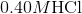.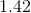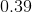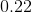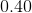Explanation:

Since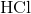is a strong acid, the concentration of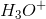is equal to the concentration of the acid itself.

Thus,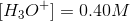.

Recall how to find the pH of a solution: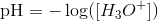Plug in the given hydronium ion concentration to find the pH of the given solution.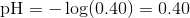Remember to maintain the correct number of significant figures.

### Example Question #2 : P H

Find the pH for a solution that is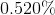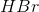by mass. Assume a density of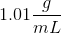for the solution.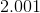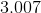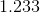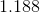Explanation:

Start by assuming that there isliter of the solution. From this, we can use the given density to find the mass of the solution.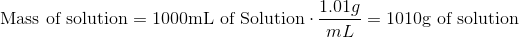Next, find the mass of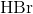that is present in the solution.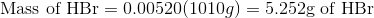Now, find the number of moles ofthat is present in the solution.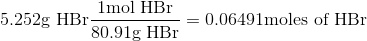Since we initially assumed that we hadliter of the solution, we now also know the concentration ofin this solution.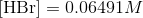Sinceis a strong acid, the concentration of hydronium ions in the solution will be the same as the concentration of.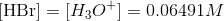Recall how to find the pH of a solution.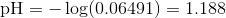### Example Question #3 : P H

Find the pH of a solution that is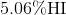by mass. Assume a density offor the solution.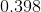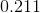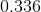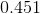Explanation:

Start by assuming that there isliter of the solution. From this, we can use the given density to find the mass of the solution.Next, find the mass of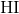that is present in the solution.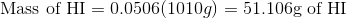Now, find the number of moles ofthat is present in the solution.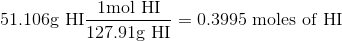Since we initially assumed that we hadliter of the solution, we now also know the concentration ofin this solution.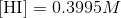Sinceis a strong acid, the concentration of hydronium ions in the solution will be the same as the concentration of.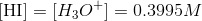Recall how to find the pH of a solution.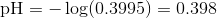### Example Question #4 : P H

Which of the following techniques will decrease the pH of a solution?

Increasing the concentration of protons

Adding more acid at the same Molarity of the solution

Increasing the amount of solvent

Decreasing the concentration of protons

Increasing the amount of Hydroxide molecules